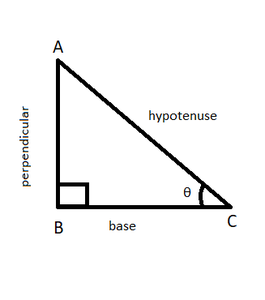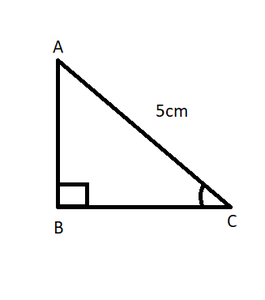If sin θ = 12/13, find the value of sin² θ- cos² θ/2 sin θ.cos θ x 1/tan² θ

• Last Updated : 22 Sep, 2021

Trigonometry is basically the study of the relationship between the angles and the sides of a triangle. It is one of the widely used topics of Mathematics that is used in daily life. It involves operations on a right-angled triangle i.e. a triangle having one of the angles equal to 90°. There are some terms that we should know before going further. These terms are,

1. Hypotenuse – it is the side opposite to the right angle in a right-angled triangle. It is the longest side of a right-angled triangle. In Figure 1, side AC is the hypotenuse.
2. Perpendicular – the perpendicular of a triangle, corresponding to a particularly acute angle θ is the side opposite to the angle θ. In Figure 1, side AB is the perpendicular corresponding to angle θ.
3. Base – it is the side adjacent to a particularly acute angle θ. In Figure 1 side BC is the base corresponding to angle θ.Figure 1

As earlier said, trigonometry depicts the relationship between the angles and sides of a right-angled triangle. These relationships is represented by standard ratios and are given as follows,

• Sine (sin) The sine of an angle θ is the ratio of the length of the perpendicular, corresponding to the angle θ, to the length of the hypotenuse of the triangle.

sin θ = perpendicular/hypotenuse = p/h

• Cosine (cos) The cosine of an angle θ is the ratio of the length of the base, corresponding to the angle θ, to the length of the hypotenuse of the triangle.

cos θ = base/hypotenuse = b/h

• Tangent (tan) The tangent of an angle θ is the ratio of the length of the perpendicular, corresponding to the angle θ, to the length of the base for the particular angle of the triangle.

tan θ = perpendicular/base = p/b

• Cotangent (cot) It is the reciprocal of a tangent.

cot θ = 1/tan θ=base/perpendicular = b/p

• Secant (sec) It is the reciprocal of cosine.

sec θ =1/cos θ = hypotenuse/base = h/b

• Cosecant (cosec) It is the reciprocal of sine.

cosec θ = 1/sin θ = hypotenuse/perpendicular = h/p

There also exist relations between each of these ratios and some of them that we will be using are,

• tan θ = sin θ/ cos θ
• cot θ = cos θ/ sin θ
• sin² θ + cos² θ =1

If sin θ = 12/13, 0° < θ < 90°, find the value of sin² θ – cos² θ /2 sin θ × cos θ × 1/tan² θ

Solution:

Given,

sin θ =12/13

sin² θ = 144/169

It is known,

sin² θ + cos² θ = 1

cos² θ = 1 – sin² θ

cos θ = √(1 – sin² θ)

Here, sin θ = 12/13

Therefore,

cos θ = √(1 – (12/13)2)

cos θ = √(1 – 144/169)

cos θ = √((169 – 144)/169)

cos θ = √(25/169)

cos θ = 5/13

and cos² θ = 25/169

tan θ = sin θ/cos θ

tan θ = (12/13)/(5/13)

tan θ = (12/13) × (13/5)

tan θ = 12/5

tan2 θ = 144/25

With all these in our hands, now find the value of our equation

(sin² θ – cos² θ )/(2 sin θ × cos θ ) × 1/tan² θ

= (144/169 – 25/169)/(2 × 12/13 × 5/13) × 1/(144/25)

= (119/169) / (120/169) × (25/144)

= (119/169) × (169/120) × (25/144)

= 0.172

Therefore, the required answer of the given equation is 0.172.

Similar Problems

Question 1: If sin θ = 1/2 and cos ϕ = √3/2 then find the value of (tan θ + tan  ϕ) /(1- tan θ × tan  ϕ).

Solution:

sin θ = 1/2

sin² θ + cos² θ = 1

cos² θ = 1 – sin² θ

cos θ = √(1 – sin² θ)

cos θ = √(1 – 1/4)

cos θ = √3/2

tan θ = sin θ / cos θ

= (1/2)/(√3/2)

= 1/√3

Similarly,

sin ϕ = √(1 – cos2  ϕ)

= √(1 – 3/4)

= 1/2

tan ϕ  = sin ϕ / cos ϕ

= (1/2)/(√3/2)

= 1/√3

Therefore,

(tan θ + tan  ϕ) /(1- tan θ × tan  ϕ) = (1/√3 + 1/√3)/( 1 -1/√3 × 1/√3)

= (2/√3)/(1 – 1/3)

= (2/√3)/(2/3)

= (2/√3) × (3/2)

= √3

Question 2: If 5 cos x – 12 sin x = 0 then find the value of (sin x + cos x)/(2 cos x – sin x).

Solution:

5 cos x – 12 sin x =0

5 cos x = 12 sin x

5 = 12 × sinx/cos x

5 = 12 tanx

tan x = 5/12

(sin x + cos x)/(2 cos x – sin x)

dividing the numerator and denominator by cos x,

(tan x + 1)/(2 – tan x)

= (5/12 + 1)/(2 – 5/12)

= (17/12)/(19/12)

= 17/19

Question 3: If a cos x + b sin x= t and a sin x – b cos x = u, then find sin x and cos x.

Solution:

Given,

a cos x + b sin x = t   ⇢  (i)

a sin x – b cos x = u  ⇢  (ii)

By, b × (i) + a × (ii),

ab cos x + b2 sin x + a2 sin x – ab cos x = bt + a

sin x (a2 + b2)= bt + au

sin x = (bt + au)/(a2 + b2)

Similarly,

By, a × (i) – b × (ii), we get

a2 cos x + ab sin x – ab sin x + b2 cos x = at – bu

cos x (a2 + b2) = at – bu

cos x = (at – bu)/(a2 + b2)

Question 4: In the right-angled triangle ABC, angle B = 90° and tan C = 1/2. If AC = 5, find the lengths of the side AB and BC.Solution:

Given, tan C = 1/2

tan C = p/b = AB/BC

Therefore,

tan C = AB/BC =1/2

Let, AB and BC be k and 2k respectively.

By Pythagoras’ theorem,

AB2 + BC2 = AC2

k2 + (2k)2 = 52

5 k2= 25

k2= 5

k = √5

Therefore,

AB = k = √5 and

BC = 2k = 2√5

My Personal Notes arrow_drop_up